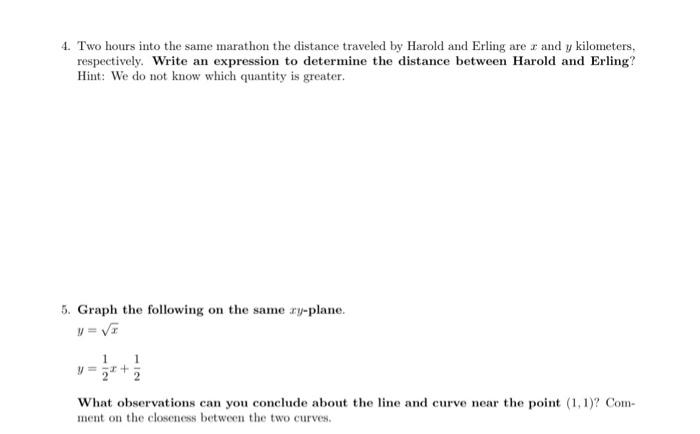Home / Expert Answers / Precalculus / 4-two-hours-into-the-same-marathon-the-distance-traveled-by-harold-and-erling-are-x-and-y-pa746

# (Solved): 4. Two hours into the same marathon the distance traveled by Harold and Erling are $$x$$ and $$y ...4. Two hours into the same marathon the distance traveled by Harold and Erling are \( x$$ and $$y$$ kilometers, respectively. Write an expression to determine the distance between Harold and Erling? Hint: We do not know which quantity is greater. 5. Graph the following on the same $$x y$$-plane. $\begin{array}{l} y=\sqrt{x} \\ y=\frac{1}{2} x+\frac{1}{2} \end{array}$ What observations can you conclude about the line and curve near the point $$(1,1)$$ ? Comment on the closeness between the two curves.

We have an Answer from Expert